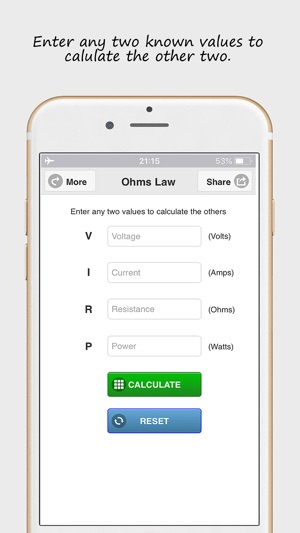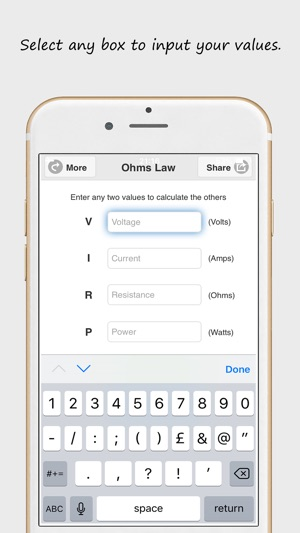## Screenshots

•••## Description

Ohms Law calculator for calculating V I R formulas. Enter any two known values to calculate the other two.

V = Voltage
I = Current
R = Resistance
P = Power

An Ohm's Law calculator to calculate V, I, R & P (Voltage, Current, Resistance & Power)

V = Voltage measured in Volts
I = Current measured in Amps
R = Resistance measured in Ohms
P = Power measured in Watts

Ohm's law states that the electrical current through a conductor between two points is directly proportional to the voltage across the two points. Introducing the constant of proportionality, the resistance, one arrives at the usual mathematical equation that describes this relationship.

Check out our suite of electrical apps @ http://www.procertssoftware.com/apps

## What’s New

Version 1.3

Thank you for downloading Ohms Law calculator. This update brings new UI improvements and bug fixes.

5.0 out of 5
2 Ratings

2 Ratings

Handy little app

## Information

Provider
Pro Certs Software Ltd
Size
7.9 MB
Category
Utilities
Compatibility

Requires iOS 8.0 or later. Compatible with iPhone, iPad and iPod touch.

Languages

English

Age Rating
Rated 4+
© Pro Certs Software Ltd
Price
Free

## Supports

•